Open in App
Not now

# Class 10 NCERT Solutions- Chapter 10 Circles – Exercise 10.2

• Difficulty Level : Easy
• Last Updated : 05 May, 2021

Theorem 10.1(NCERT) : The tangent at any point of a circle is perpendicular to the radius through the point of contact.

Theorem 10.2 (NCERT) : The lengths of tangents drawn from an external point to a circle are equal.

### Question 1. From a point Q, the length of the tangent to a circle is 24 cm and the distance of Q from the centre is 25 cm. The radius of the circle is

(A) 7 cm

(B) 12 cm

(C) 15 cm

(D) 24.5 cm

Solution: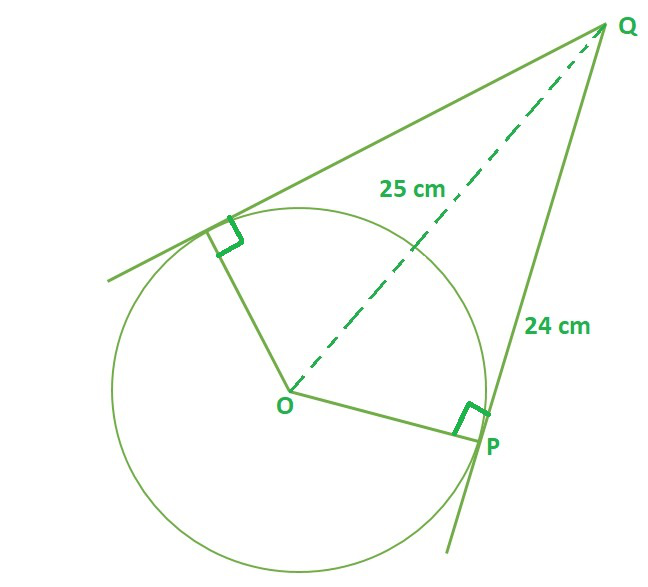According to theorem 10.1, OP⊥ PQ then ∆OPQ is a right-angled triangle

OQ2 = PQ2 + OP2  (Pythagoras Theorem)

252 = 242 + OP

OP2 = 252 – 24

OP2 = (25+24) (25-24)                               (using identity a2 – b2 = (a+b)(a-b))

OP = √49

OP = 7 cm

Hence, option A is correct.

### Question 2. In Fig. 10.11, if TP and TQ are the two tangents to a circle with centre O so that ∠ POQ = 110°, then ∠ PTQ is equal to

(A) 60°

(B) 70°

(C) 80°

(D) 90°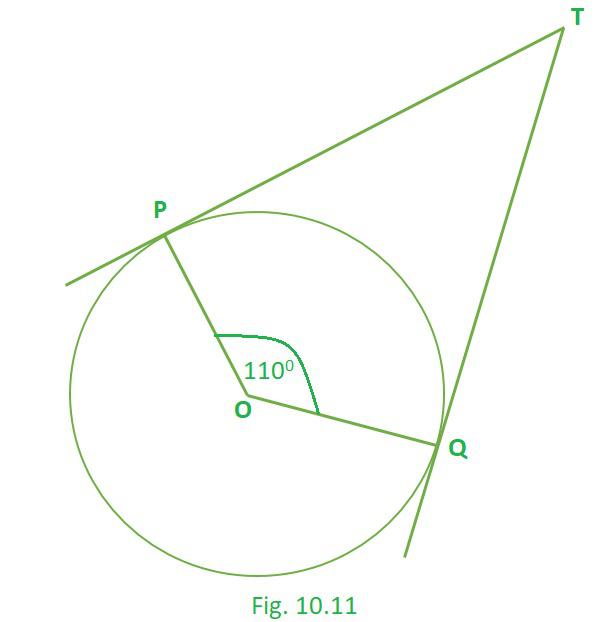Solution:

∠P = 90°, ∠Q = 90°  (Theorem 10.1)

∠O = 110°

The sum of the angles of a quadrilateral is 360° (Angle sum property of quadrilateral), Hence

∠P + ∠Q + ∠T + ∠O = 360°

90° + 90° + ∠T + 110° = 360°

∠T = 180 – 110° = 70°

Hence, option B is correct.

### Question 3. If tangents PA and PB from a point P to a circle with centre O are inclined to each other at angle of 80°, then ∠ POA is equal to

(A) 50°

(B) 60°

(C) 70°

(D) 80°

Solution: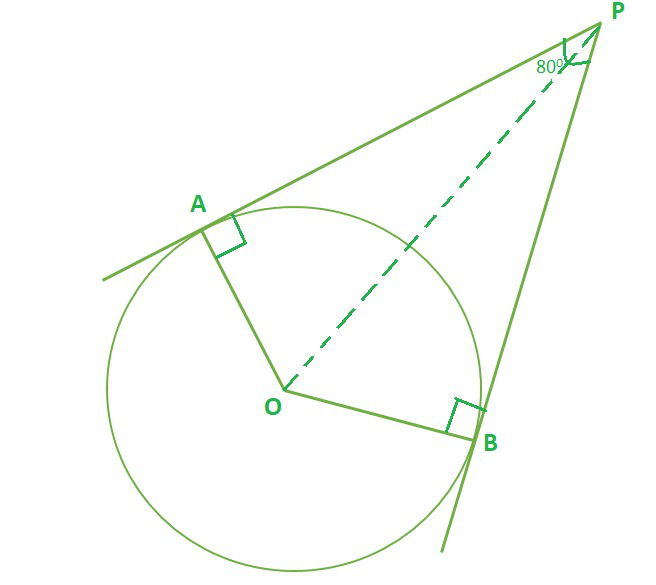∠A = 90°, ∠B = 90°  (Theorem 10.1)

∠P = 80°

The sum of the angles of a quadrilateral is 360° (Angle sum property of quadrilateral), Hence

∠A + ∠B + ∠P + ∠O = 360°

90° + 90° + 80° + ∠O = 360°

∠O = 180° – 80° = 100° …………………..(1)

Considering, ∆OAP and ∆OBP

OA = OB …………..(radius of circle)

AP = BP ……………(Theorem 10.2)

∠OAP = ∠OBP …….(Theorem 10.1)

∴ ∆OAP ≅ ∆OBP           [By SAS congruency]

So, ∠AOP = ∠BOP [By C.P.C.T.]…………..(2)

From (1) and (2), we conclude that,

∠AOP + ∠BOP = 100°

∠AOP = 50°

Hence, option A is correct.

### Question 4. Prove that the tangents drawn at the ends of a diameter of a circle are parallel.

Solution:

P and Q are point of contacts of Tangent lines l and m respectively.

O is the centre of circle.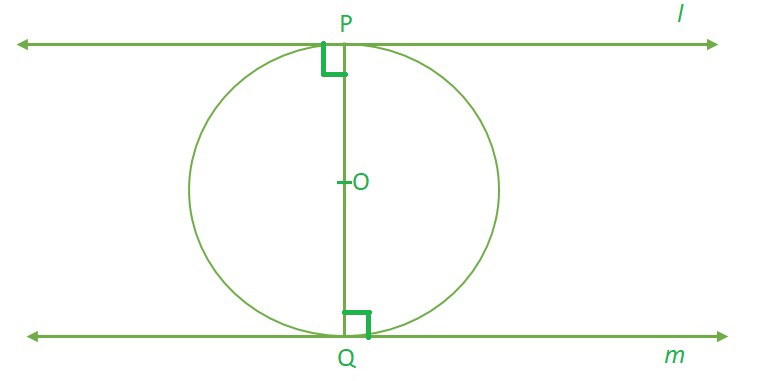OP⊥ l , OQ⊥ m and PQ is diameter (theorem 10.1)

∠PQm + ∠QPl = 90° + 90° = 180°

As, sum of adjacent angles is supplementary (180°), hence opposite sides are parallel.

### Question 5. Prove that the perpendicular at the point of contact to the tangent to a circle passes through the centre.

Solution:

P is the point of contact of tangent line l.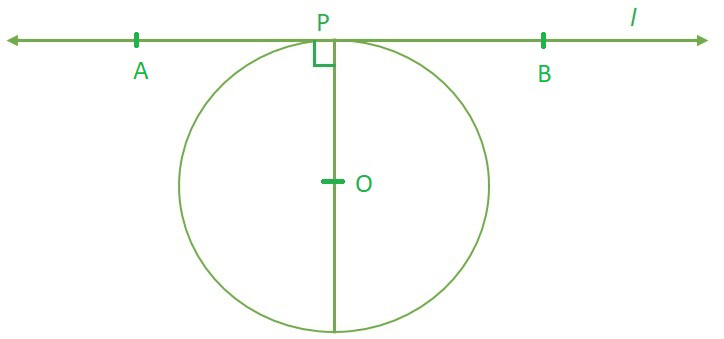Let, OP⊥ l at Point of contact P and it passes through point O.

As, The tangent at any point of a circle is perpendicular to the radius through the point of contact. (theorem 10.1)

According to the theorem 10.1 line OP has to pass through centre of circle for sure.

### Question 6. The length of a tangent from a point A at distance 5 cm from the centre of the circle is 4 cm. Find the radius of the circle.

Solution: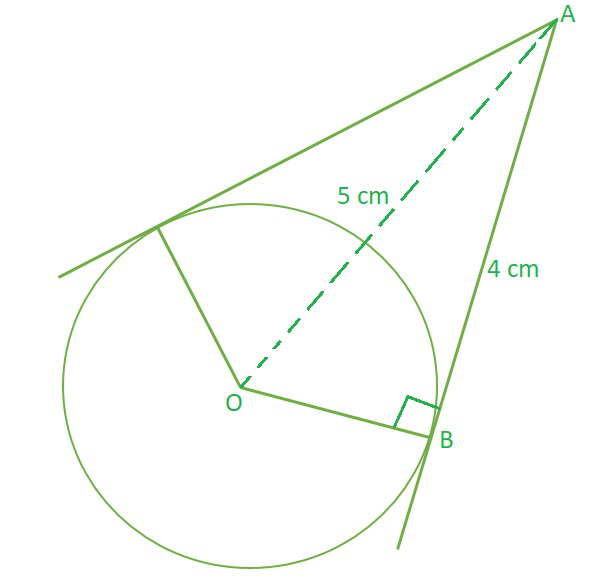According to theorem 10.1, OB⊥ AB then ∆OAB is a right-angled triangle

OA2 = AB2 + OB2  (Pythagoras Theorem)

52 = 42 + OB2

OB2 = 52 – 42

OB2 = (5+4) (5-4)                               (using identity a2 – b2 = (a+b)(a-b))

OB = √9

OB = 3 cm

Hence, Radius of circle = 3 cm

### Question 7. Two concentric circles are of radii 5 cm and 3 cm. Find the length of the chord of the larger circle which touches the smaller circle.

Solution: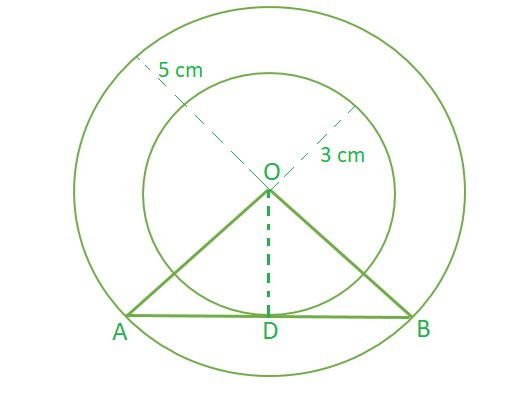OA = OB …………..(radius of large circle)

OD = OD ……………(common side)

∠ADO = ∠BDO …….(each 90°)…….(Theorem 10.1)

∴ ∆OAD ≅ ∆OBD           [By SAS congruency]

So, AD = BD [By C.P.C.T.]…………….(1)

Taking ∆OAD, which 90° at ∠D

OA = OB = 5 cm (radius of large circle)

OD = 3 cm  (radius of smaller circle)

OA2 = AD2 + OD2  (Pythagoras Theorem)

AB = 2 × AD (from 1)

AB = 2 × 4

AB = 8 cm

Hence, length of the chord of the larger circle which touches the smaller circle = 8 cm

### Question 8. A quadrilateral ABCD is drawn to circumscribe a circle (see Fig. 10.12). Prove that AB + CD = AD + BC.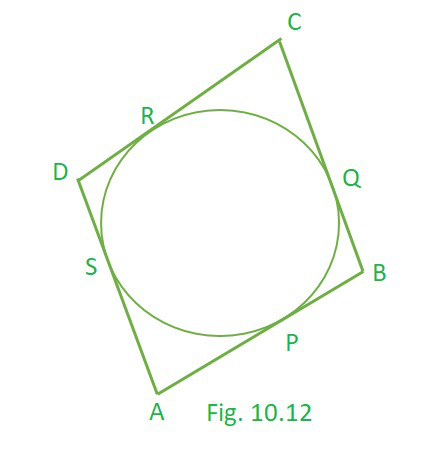Solution:

Let the P, Q, R and S be point of contacts for tangent AB, BC, CD and DA respectively .

AP = AS  (theorem 10.2)……..(1)

BP = BQ  (theorem 10.2)……..(2)

CR = CQ  (theorem 10.2)……..(3)

DR = DS  (theorem 10.2)……..(4)

By, adding (1), (2), (3) and (4) RHS = LHS, we get

AP + BP + CR + DR = AS + BQ + CQ + DS

(AP + BP) + (CR + DR) = (AS + DS) + (BQ + CQ)  (by Rearranging)

AB + CD = AD + BC

Hence, proved !!

### Question 9. In Fig. 10.13, XY and X′Y′ are two parallel tangents to a circle with centre O and another tangent AB with point of contact C intersecting XY at A and X′Y′ at B. Prove that ∠ AOB = 90°.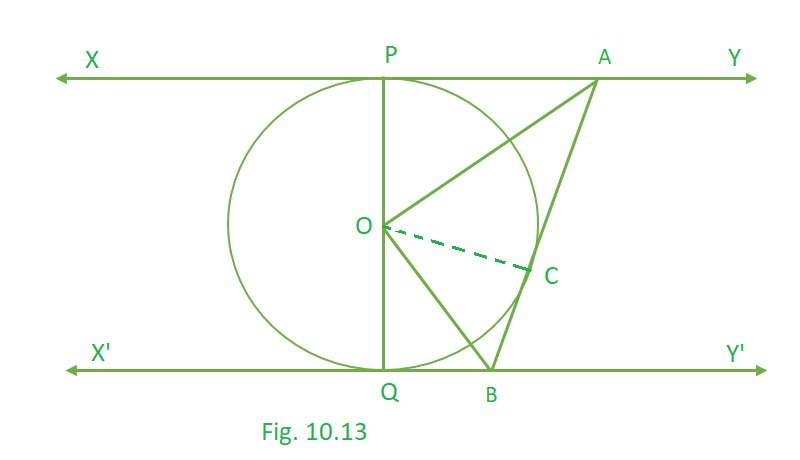Solution:

As we can observe here,

AP and AC are tangent at same external point A.

and, QB and BC are tangent at same external point B.

Taking, ∆OAP and ∆OAC in consideration

OP = OC …………..(radius of circle)

OA = OA ……………(common side)

∠OPA = ∠OCA …….(each 90°)…….(Theorem 10.1)

∴ ∆OAP ≅ ∆OAC           [By SAS congruency]

So, ∠POA = ∠COA [By C.P.C.T.]

we can conclude that, ∠COP = 2 ∠COA………………..(1)

Similarly, ∠COQ = 2 ∠COB ………….(2)

Adding (1) and (2), RHS = LHS we get,

2 ∠COA + 2 ∠COB = ∠COQ + ∠COP

2 (∠COA + ∠COB) = 180°  (Angle made by a straight line = 180°)

2 (∠AOB) = 180°

∠AOB = 90°.

Hence, proved !!

### Question 10. Prove that the angle between the two tangents drawn from an external point to a circle is supplementary to the angle subtended by the line segment joining the points of contact at the Centre.

Solution: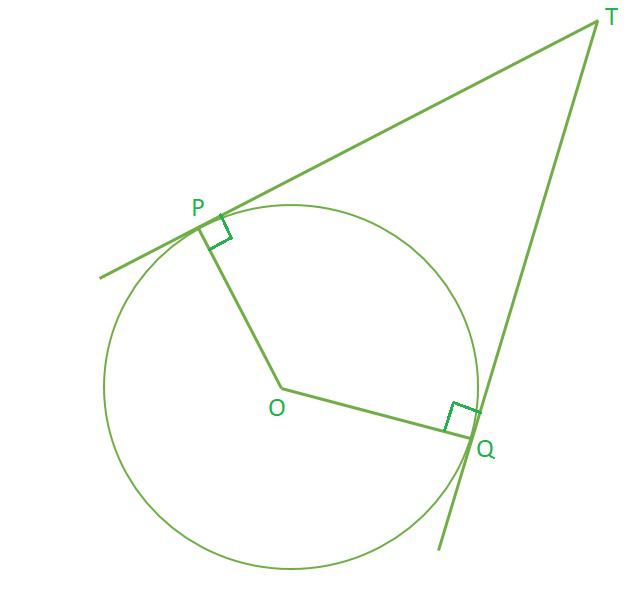∠P = 90°, ∠Q = 90°  (Theorem 10.1)

The sum of the angles of a quadrilateral is 360° (Angle sum property of quadrilateral), Hence

∠P + ∠Q + ∠T + ∠O = 360°

90° + 90° + ∠T + ∠O = 360°

∠T + ∠O = 180°

Hence, Proved, the angle between the two tangents drawn from an external point to a circle is supplementary to the angle subtended by the line segment joining the points of contact at the Centre.

### Question 11. Prove that the parallelogram circumscribing a circle is a rhombus.

Solution:

ABCD is a parallelogram and let P, Q, R and S be the point of contact of circle and parallelogram.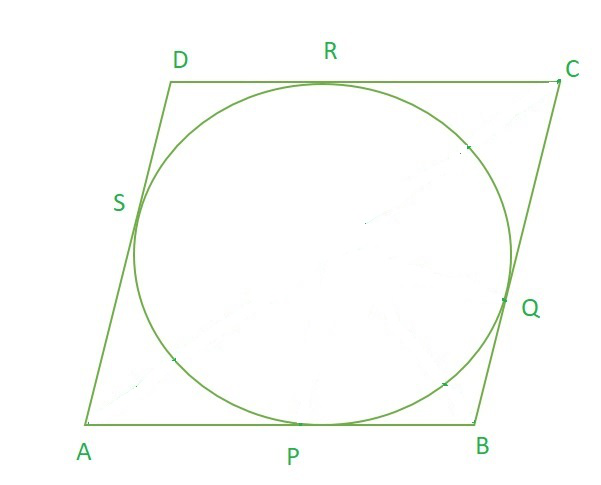AP = AS  (theorem 10.2)……..(1)

BP = BQ  (theorem 10.2)……..(2)

CR = CQ  (theorem 10.2)……..(3)

DR = DS  (theorem 10.2)……..(4)

By, adding (1), (2), (3) and (4) RHS = LHS, we get

AP + BP + CR + DR = AS + BQ + CQ + DS

(AP + BP) + (CR + DR) = (AS + DS) + (BQ + CQ)  (by Rearranging)

AB + CD = AD + BC

As ABCD is a parallelogram, AB = CD and AD = BC (Opposite sides of parallelogram are equal)

Hence, 2 AB = 2 BC

AB = BC

If adjacent side of parallelogram are equal, then it is a rhombus.

Hence, ABCD is a rhombus !!

### Question 12. A triangle ABC is drawn to circumscribe a circle of radius 4 cm such that the segments BD and DC into which BC is divided by the point of contact D are of lengths 8 cm and 6 cm respectively (see Fig. 10.14). Find the sides AB and AC.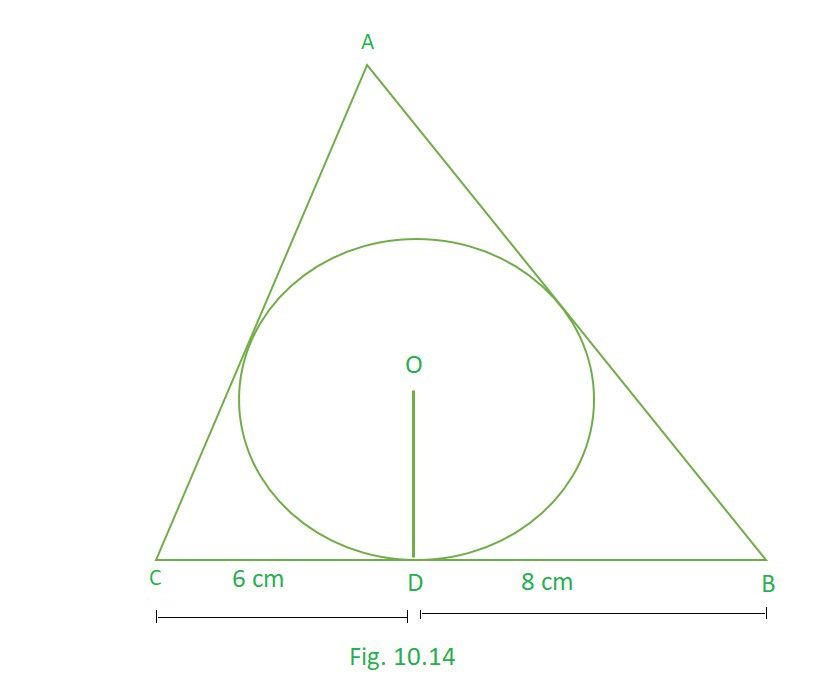Solution:

ABC is a Triangle and let M, D and N be the point of contact of circle and Triangle.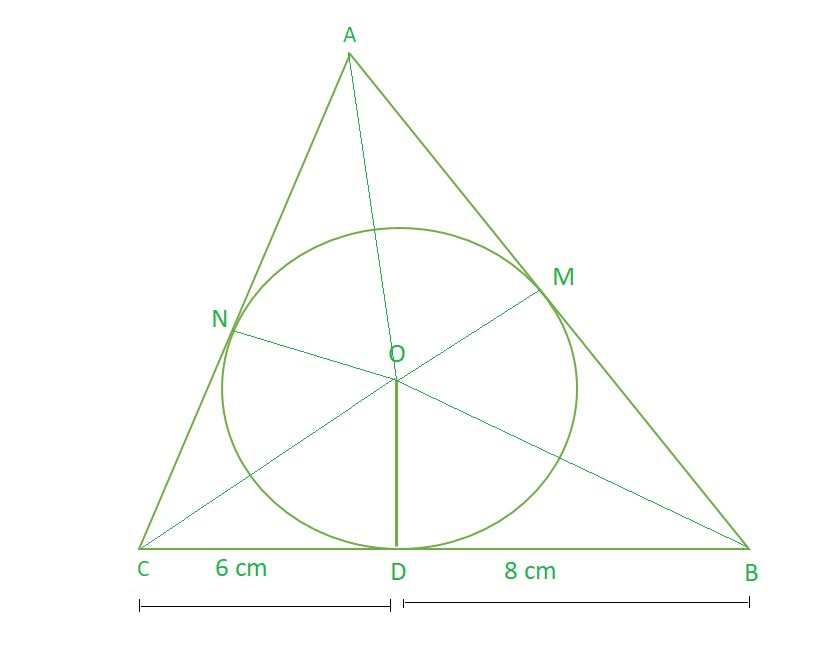BD = BM =  8 cm (theorem 10.2)……..(1)

CN = CD  = 6 cm (theorem 10.2)……..(2)

AN = AM  = p cm (theorem 10.2)……..(3)

AB = p+8 cm

BC = 6+8 = 14 cm

AC = p+6 cm

As we can observe here that,

Area of ∆ABC = Area of ∆AOC + Area of ∆COB + Area of ∆BOA

So, Area of ∆ABC = ar(∆ABC)

√(s (s-AB) (s-AC) (s-BC))     …………(Heron’s formula)  where s = (sum of sides) / 2

s = (AB+BC+AC)/2

s = (p+8+14+p+6)/2

s = (2p+28)/2

s = p+14

ar(∆ABC) = √((p+14) (p+14-(p+8)) (p+14-(p+6)) (p+14-14))

= √((p+14) (6) (8) (p))

= √48p (p+14) cm2……………………………………………(1)

Area of ∆AOC + Area of ∆COB + Area of ∆BOA = (½ × ON × AC) + (½ × OD × BC) + (½ × OM × AB)

= (½ × 4 × (p+6)) + (½ × 4 × 14) + (½ × 4 × (p+8))

= ½ × 4 (p+6+14+p+8)

= ½ × 4 × (2p+28)

= 4 × (p+14) cm2  ………………………………………………(2)

(1) = (2)

√48p (p+14) =  4 × (p+14)

Squaring both sides, we get

48 × p × (p+14) = (4 × (p+14))2

48 × p (p+14) = 16 × (p+14)2             (cancelling (p+14) from both sides)

48 × p = 16 (p+14)

48p = 16p+ 224

32 × p = 224

p = 7 cm

Hence, AB = p+8 = 7+8 = 15 cm

AC = p+6 = 7+6 = 13 cm

### Question 13. Prove that opposite sides of a quadrilateral circumscribing a circle subtends supplementary angles at the Centre of the circle.

Solution: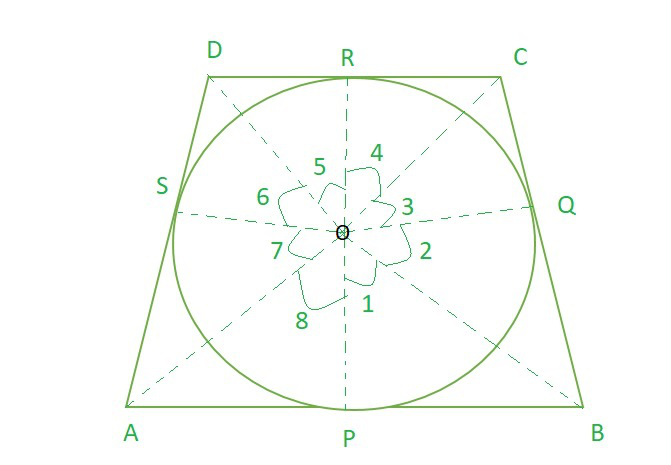∠OPB = 90° , ∠OQB = 90°  (Theorem 10.1)

Considering, ∆OPB and ∆OQB

OP = OQ …………..(radius of circle)

OB = OB ……………(Common)

∠OPB = ∠OQB …….(each 90°)……..(Theorem 10.1)

∴ ∆OPB ≅ ∆OQB           [By SAS congruency]

So, ∠POB = ∠QOB [By C.P.C.T.]

Hence, ∠1 = ∠2  ……………..(1)

Similarly, ∠3 = ∠4  ……………..(2)

∠5 = ∠6  ……………..(3)

∠7 = ∠8  ……………..(4)

By making complete revolution,

∠1 + ∠2 + ∠3 + ∠4 + ∠5 + ∠6 + ∠7 + ∠8 = 360° (A complete revolution makes 360°)

∠1 + ∠1 + ∠4 + ∠4 + ∠5 + ∠5 + ∠8 + ∠8 = 360°

2 (∠1 + ∠4 + ∠5 + ∠8) = 360°

2 ((∠1 + ∠8) + (∠4 + ∠5) ) = 360°

∠AOB + ∠COD = 360° / 2 = 180°

Hence, proved, that opposite sides of a quadrilateral circumscribing a circle subtend supplementary angles at the Centre of the circle.

My Personal Notes arrow_drop_up